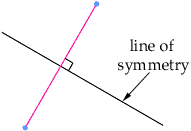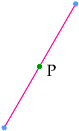Name: Sonam Who is asking: Student Level: Secondary Question: What is symmetry? Who invented symmetry? what are the different kinds of symmetry? Hi Sonam, You should use a dictionary to find a definition of the word symmetry. A geometric figures in the plane can have symmerty in a line or symmetry in a point. Two points in the plane are symmetric in the line of symmetry L if the two points are the same distance from L and the line joining them is perpendicular to L.Two points in the plane are symmetric in a point P if the midpoint of the line joining the points.I hope this helps Penny Go to Math Central